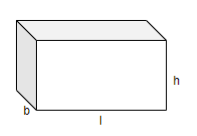QUESTION

# How many bricks, each measuring $25cm\times 11.25cm\times 6cm$, will be needed to build a wall $8m\times 6m\times 22.5cm$?

Hint: Calculate the volume of each brick using the fact that the volume of a cuboid with length ‘l’, breadth ‘b’, and height ‘h’ is given by ‘lbh’. Calculate the volume of the wall using the same formula. Divide the volume of the wall by the volume of brick to calculate the number of bricks needed to build a wall.

We have to calculate the number of bricks, each of dimensions $25cm\times 11.25cm\times 6cm$ that can be used to make a wall of dimensions $8m\times 6m\times 22.5cm$.
We will first calculate the volume of brick and wall.
We know that the volume of a cuboid with length ‘l’, breadth ‘b’, and height ‘h’ is given by lbh.We will now calculate the volume of brick. We know that the dimensions of the brick are $25cm\times 11.25cm\times 6cm$.
Substituting $l=25cm,b=11.25cm,h=6cm$ in the above expression, the volume of brick is $=25cm\times 11.25cm\times 6cm=1687.5c{{m}^{3}}$.
We will now calculate the volume of the wall. We know that the dimensions of the wall are $8m\times 6m\times 22.5cm$. We will firstly convert these dimensions into centimetres.
We know that $1m=100cm$. To calculate the value of x m, we will multiply ‘x’ by 100.
Thus, we can rewrite 8m as $8m=8\times 100=800cm$.
Similarly, we can rewrite 6m as $6m=6\times 100=600cm$.
Thus, the dimensions of the wall is $800cm\times 600cm\times 22.5cm$. We will now calculate the volume of the wall.
Thus, the volume of the wall is $=800cm\times 600cm\times 22.5cm=10,800,000c{{m}^{3}}$.
To calculate the number of bricks needed to make the wall, we will divide the volume of the wall by the volume of bricks.
Thus, the number of bricks needed $=\dfrac{10800000}{1687.5}=6400$.
Hence, we need 6400 bricks, each of dimensions $25cm\times 11.25cm\times 6cm$ to make the wall of dimensions $8m\times 6m\times 22.5cm$.

Note: It’s necessary to have the same dimensions of wall and bricks. We can also solve this question by converting all the measurements to metres and then calculating the volume. If we don’t keep the dimensions the same, we will get an incorrect answer.